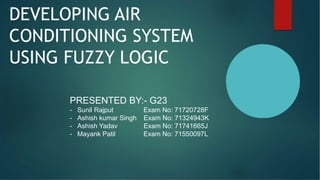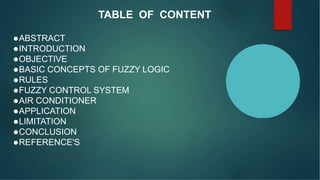Successfully reported this slideshow.

# DEVELOPING Air Conditioner Controller using MATLAB Fuzzy logic presentation

0

Share×
1 of 20
1 of 20

# DEVELOPING Air Conditioner Controller using MATLAB Fuzzy logic presentation

0

Share

Solve Air Conditioner Controller using MATLAB Fuzzy logic toolbox or Octave or Python.

Solve Air Conditioner Controller using MATLAB Fuzzy logic toolbox or Octave or Python.

## More Related Content

### Related Books

Free with a 14 day trial from Scribd

See all

### Related Audiobooks

Free with a 14 day trial from Scribd

See all

### DEVELOPING Air Conditioner Controller using MATLAB Fuzzy logic presentation

1. 1. Department of Computer Engineering Sandip Foundation's Sandip Institute of Technology and Research Centre, Nashik Savitribai Phule Pune University BE PROJECT Year 2019 – 2020 Under the Guidance Prof. Vivek Waghmare
2. 2. DEVELOPING AIR CONDITIONING SYSTEM USING FUZZY LOGIC PRESENTED BY:- G23 - Sunil Rajput Exam No: 71720728F - Ashish kumar Singh Exam No: 71324943K - Ashish Yadav Exam No: 71741665J - Mayank Patil Exam No: 71550097L
3. 3. TABLE OF CONTENT ABSTRACT INTRODUCTION OBJECTIVE BASIC CONCEPTS OF FUZZY LOGIC RULES FUZZY CONTROL SYSTEM AIR CONDITIONER APPLICATION LIMITATION CONCLUSION REFERENCE'S
4. 4. ABSTRACT Fuzzy logic control was developed to control the compressor motor speed , fan speed , fin direction and operation mode to maintain the room temperature at or closed to the set point temperature and save energy and keep devices from damage. This paper describes the development of Fuzzy logic algorithm for Air Condition control system. This system consists of four sensors for feedback control: first for input electric volt which used to save devices from damage due to alternated voltages, second for temperature and third for humidity and fourth for dew point. Simulation of the Fuzzy logic algorithm for Air Condition controlling system is carried out based on MATLAB.
5. 5. INTRODUCTION First proposed in 1965 by Lotfi Zadeh as a way to process imprecise data. • Fuzzy Logic (FL) controlling system is based on a set of rules established by an expert. • These rules are translated into mathematical steps used to realize a physical controller. • FL controllers can be physically realized in different forms. • We adopt look up tables and function realizations Lotfi Aliasker Zadeh
6. 6. muruganm1@gmail.com • Instead of using complex mathematical equations fuzzy logic uses linguistic description to define the relationship between the input information and the output action. • Just as fuzzy logic can be described simply as “Computing with words rather than numbers”, fuzzy control can be described simply as “Control with sentences rather than equations”. What is Fuzzy Logic?
7. 7. muruganm1@gmail.com Rules :- Fuzzy logic usually uses IF-THEN rules, or constructs that are equivalent. -IF variable is property THEN action Example:- A simple temperature regulator that uses a fan might look like this: IF temperature is very cold THEN stop fan IF temperature is cold THEN turn down fan IF temperature is normal THEN maintain level IF temperature is hot THEN speed up fan
8. 8. Fuzzy Control System A fuzzy control system is based on Fuzzy Logic. The process of designing fuzzy control system can be described using following steps Step 1:Identify the principal input, output and process tasks Step 2: Identify linguistic variables used and define fuzzy sets and membershipsaccordingly Step 3: Use these fuzzy sets and linguistic variables to form procedural rules Step 4: Determine the defuzzificationmethod Step 5: Test the system and modify ifnecessary
9. 9. AirConditioner
10. 10. Controller Structure • Fuzzification – Scales and maps input variables to fuzzy sets • Inference Mechanism – Approximate reasoning – Deduces the control action • Defuzzification – Convert fuzzy output values to control signals
11. 11. Operations A B A  B A  B A
12. 12. Rule Base • Air Temperature • Set cold {50, 0, 0} • Set cool {65, 55, 45} • Set just right {70, 65, 60} • Set warm {85, 75, 65} • Set hot {, 90, 80} • Fan Speed • Set stop {0, 0, 0} • Set slow {50, 30, 10} • Set medium {60, 50, 40} • Set fast {90, 70, 50} • Set blast {, 100, 80}
13. 13. Rules in Matlab
14. 14. Rules and Membership Function via Matlab
15. 15. Fuzzy Air Conditioner 10 0 20 30 40 50 60 70 80 90 100 0 if Coldthen Stop IFCool then Slow IfJustRight the nMediu m IfWarmthenFast IfHotthen Bla st 1 4 5 5 0 5 5 6 0 6 5 7 0 7 5 8 0 0 8 5 9 0
16. 16. APPLICATIONS 1 6 WashingMachines Anti-Lock BrakingSystem Anti sway cranecontrol Flight Control in planes In Air-Conditioning Cutting force optimization in machining
17. 17. Limitations of Fuzzy Systems Fuzzy systems lack the capability of machine learning as-well-as neural network type pattern recognition Verification and validation of a fuzzy knowledge-based system require extensive testing withhardware Determining exact fuzzy rules and membership functions is a hard task Stability is an important concern for fuzzycontrol
18. 18. CONCLUSION  Fuzzy Logic provides a completelydifferent, way to approach a control problem.  Focus on what the system should dorather than trying to understand how it works.  Leads to quicker, cheapersolutions.  In case of the Air-Conditioning system, fuzzy logic helped solve a complex problem without getting involved in intricate relationships between physical variables. Intuitive knowledge about input and output parameters was enough to design an optimally performing system. With most of the problems encountered in day to day life falling in this category, like washing machines, vacuum cleaners, etc, fuzzy logic is sure to make a great impact in human life.
19. 19. • Set up the one input system as a proof of concept. We are in the process of building the hardware set up. • Based on the first system, make a selection of the microcontroller models appropriate for a two and three input system FUTURE SCOPE
20. 20. REFERENCES John Yen, Reza Langari, Fuzzy Logic Intelligence, control and Information, Prentice-Hall Inc, 1999 Ali Dr. I.M., 2012. Developing of a Fuzzy Logic Controller for Air Conditioning System, Anbar Journal for Engineering Sciences, Vol 5, 180-187. Aprea C., Mastrullu R. and Rrenno C.,2004. Fuzzy control of compressor speed in refrigerant plant, Int J Refrigerat., Vol 2, pp.134-143. Arima M., Hara E. H., and Katzberg J. D., 1995. A fuzzy logic and rough sets controller for HVAC system, IEEE WESCANEX’95, Vol 95, pp 133-138. Batayneh W., Araidah O. and Bataineh K., 2010. Fuzzy logic approach to provide safe and comfortable indoor environment, International Journal of Engineering, Science and Technology, Vol. 2, pp. 65-72. Becker M., OestreichD., Hasse Hand Litz L 1994. Fuzzy control for temperature and Humidity in refrigeration systems, IEEE transact, Vol FM-4-2, pp 1607-1611. Calvino F., Gennusa M. L., Rizzo G., 2004. The control of indoor thermal comfort conditions: introducing fuzzy adaptive controller, Ener Build, Vol 36, pp. 97-102